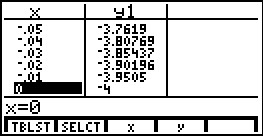# Knowledge Base

## Solution 10427: Finding the Limit of a Function Using the TI-86 Graphing Calculator.

### How do I find the limit of a function using the TI-86?

To find the limit of a function on the TI-86, it must first be graphed in the interval comprising the given value the function approaches. Then, use the table feature to display values that surround the given value. In the table setup editor, choose a beginning value that is less than the given value and the increment values to be small enough to show what the limit is.

For example, to find the limit of the function y=(x+4)/(x-1) as x approaches zero:

• Press [GRAPH] [F1] to display the equation editor.

• Enter the function next to y1=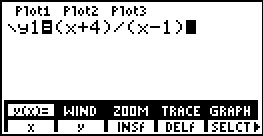• Press [2nd] [F2] to display the WINDOW menu.

• Enter the following xMin=-5, xMax=5, xScl=1, yMin=-10, yMax=10, and yScl=1.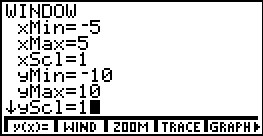• Press [F5] to display the graph.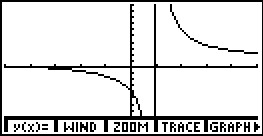• Press [TABLE] [F2] to access the table setup editor. Enter TblStart=-.1 and DTbl=0.01. Select Indpnt: Auto. The closest the TblStart value and the DTbl will be to 0, the more accurate the limit will be.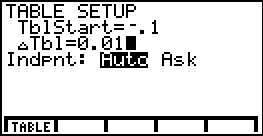• Press [F1] to display the table.

Looking at the numbers in the table, as x approaches 0, the function approaches -4. The limit of (x+4)/(x-1) as x approaches 0 is equal to -4.